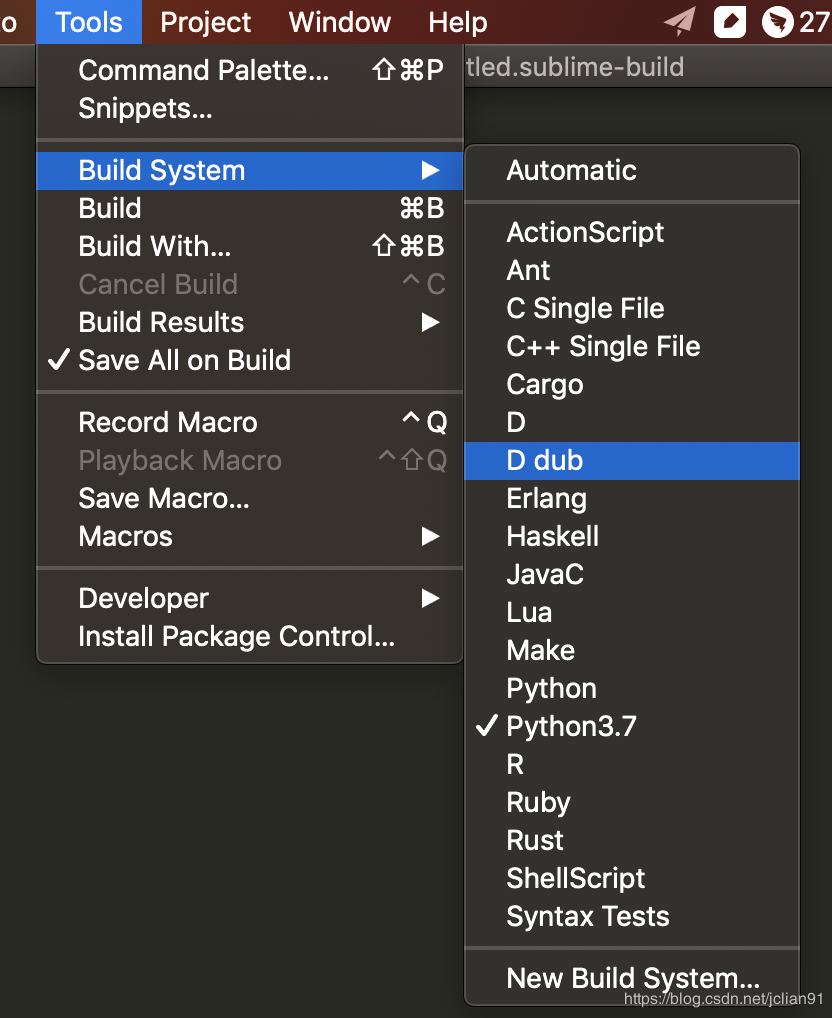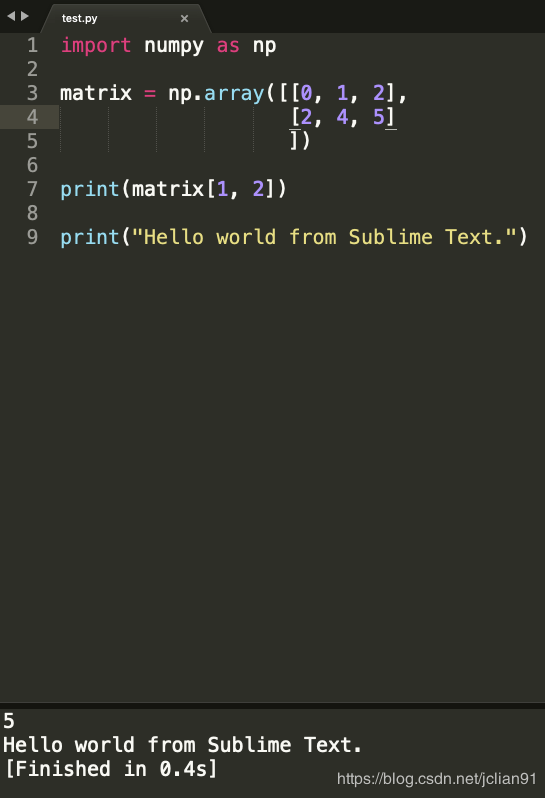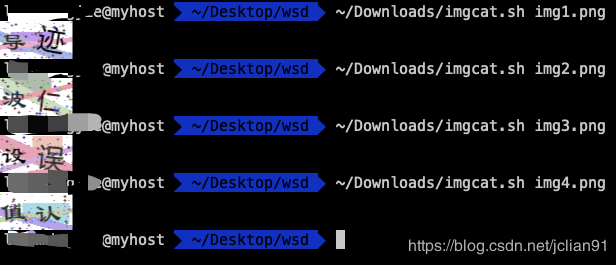# 3 个提高 Python 开发效率的小工具• 在Sublime Text中支持Python运行；
• 在Mac电脑的iTerm2中支持查看图片；
• Python的typing模块介绍。

### 在Sublime Text中支持Python运行

{
"cmd": ["D:/Anaconda3/python3.7.exe","-u","\$file"],
}import numpy as np

matrix = np.array([[0, 1, 2],
[2, 4, 5]
])

print(matrix[1, 2])

print("Hello world from Sublime Text.")### 在Mac系统的iTerm2中支持查看图片

iTerm2是Mac系统很好用的终端工具，本文不过多介绍iTerm2，而是介绍如何直接在iTerm2中查看图片，这样可以方便我们在终端直接查看图片。### Python中的typing模块介绍

typing模块是Python中提供类型支持的模块，它的主要作用为：

from typing import *

# 创建函数
def digits_sum(num:str) -> int:
digits_arr = map(lambda x: int(x), num)
return sum(digits_arr)

# 测试
num = "352"
result = digits_sum(num=num)
print(result)


from typing import Dict, Any

# 创建函数
def dict_multipy(d: Dict[str, Any]) -> Dict[str, float or int]:
new_dict = {}
for k, v in d.items():
if isinstance(v, (float, int)):
new_dict[k] = v * 2

return new_dict

# 测试
d = {"no": "100", "age": 12, "work_year": 3, "name": "JC"}
new_d = dict_multipy(d=d)
print(new_d)


from typing import List

# 取List[float]别名为Vector
Vector = List[float]

def scale(scalar: float, vector: Vector) -> Vector:
return [scalar * num for num in vector]

new_vector = scale(2.0, [1.0, -4.2, 5.4])


（版权归原作者所有，侵删）